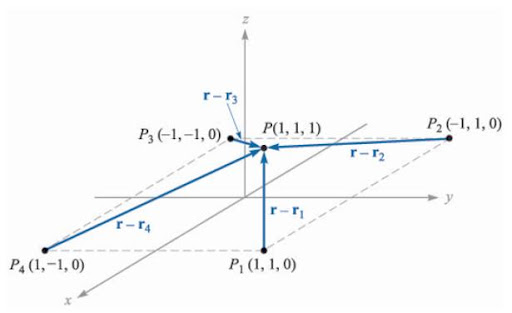# Electric Field Intensity

Consider a fixed charge Q1. Let us move a second charge say test charge Qt around the Q1. We observe that the test charge Qt experiences force everywhere around Q1. It is experiencing a force field. Force on it will be given by coulombs law as:

But still question arises what is Electric Field?? Before defining electric field let us modify above equation a bit.
Let us write the above equation as force/charge equation:

This quantity that is force/charge describes a vector quantity. This vector quantity is called Electric Field Intensity.So Electric field intesity is defined as the vector force on an unit positive test charge by a fixed charge Q1. Or it can also be defined as force per unit charge.
So to sum it up we can Electric field intensity as:

So it becomes.

Expressing Electric field in cartesian Coordinate:

Expressing Electric Field in Spderical Co-ordinates:
First for considering spherical co ordinate we must see that fixed charge has spherical symmetry at its location like at origin(0,0,0) for that we can either use spherical co-ordinate directly in equation as:

Where Er and r spherical co-ordinates values or we can convert them in cartesian coordinate which will give us the equation as below:
We will have r = R = xi + yj +zk ar = aR = (xi + yj + zk)/√(x^2+y^2+z^2 )

If more charges are added in the surrounding of Q1 then electric field will be sum of individual acting alone. As shown in equation given below:

Before ending the topic let us do a small quick example.
Example: See the figure below. We need to find out Electric field at P(1,1,1) caused by 4 identical 3nC charges located at P1(1,1,0), P2(-1,1,0), P3(-1,-1,0) and P4(1,-1,0).Example of Electric Field

Answer: As r = i + j + k and r1 = i+j therefore r – r1 = k. Therefore |r – r1| = 1.
Similaraly r – r2 = 2i + k. therefore |r – r2| = √5
Similaraly r – r3 = 2i + 2j + k. therefore |r – r3| = 3
Similaraly r – r4 = 2j + k. therefore |r – r4| = √5
And as

Therefore simplifying we get E =
E = 6.82i + 6.83j + 32.8k V/m ans.